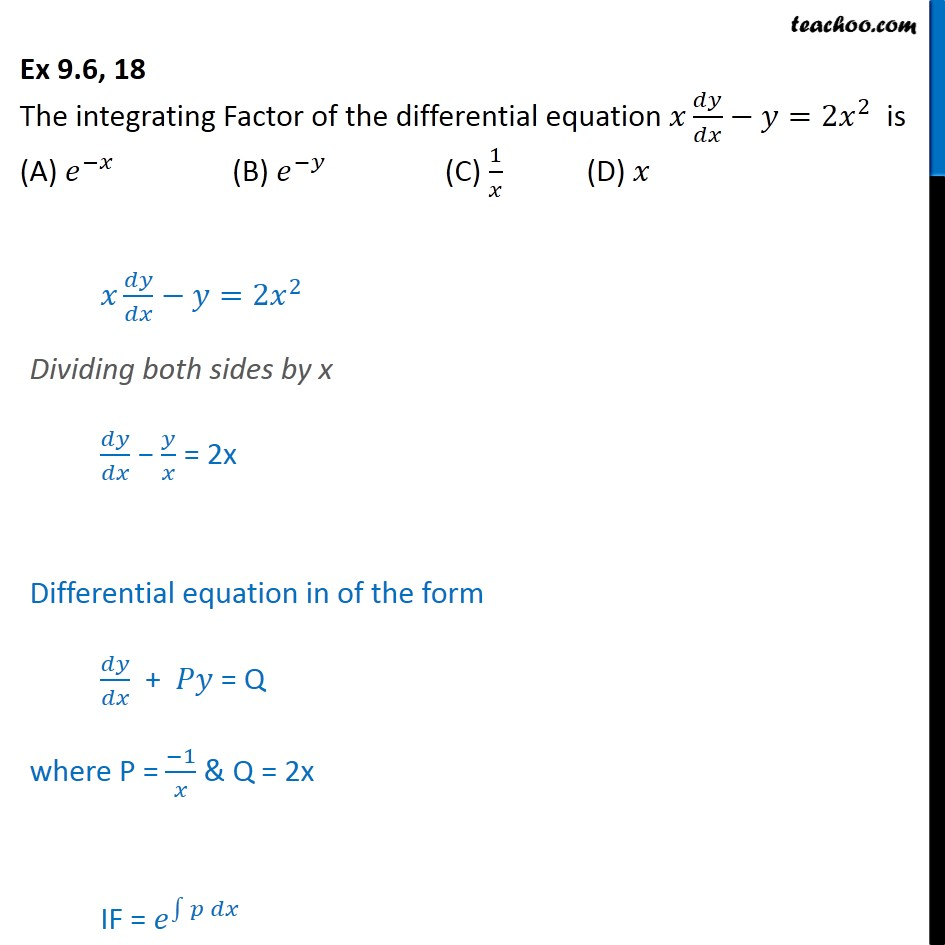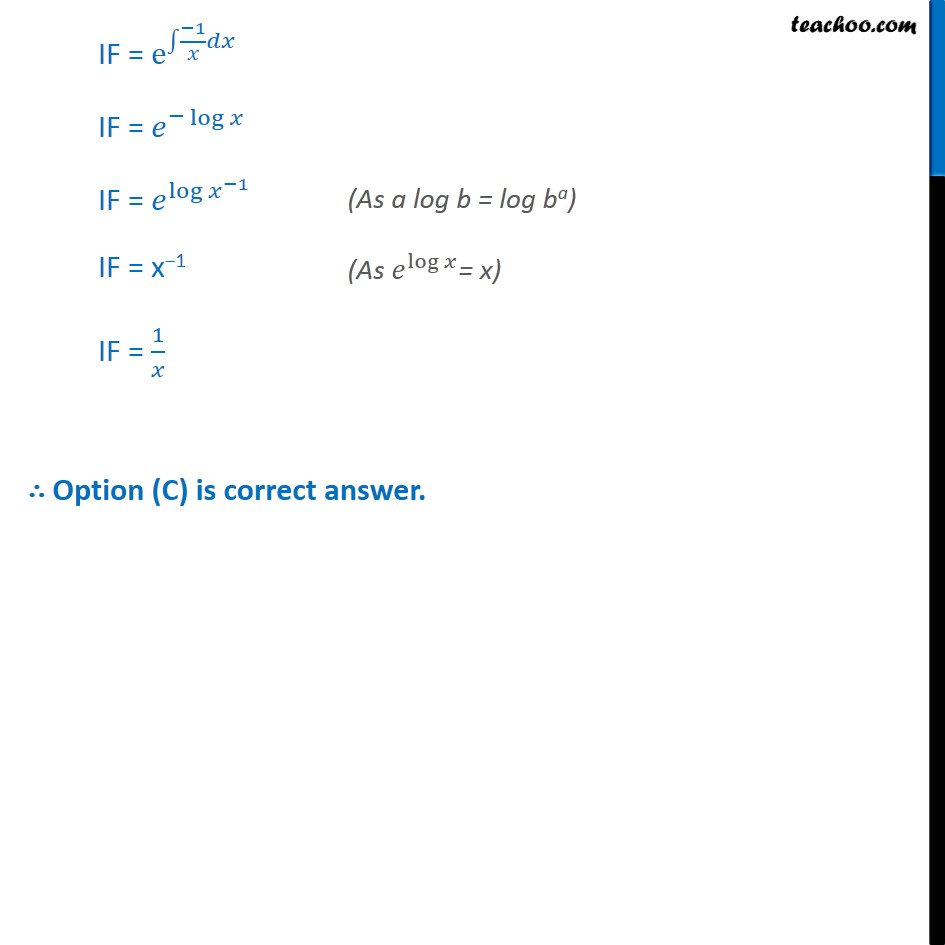Ex 9.5

Chapter 9 Class 12 Differential Equations
Serial order wiseLearn in your speed, with individual attention - Teachoo Maths 1-on-1 Class

### Transcript

Ex 9.5, 18 The integrating Factor of the differential equation =2 2 is (A) (B) (C) 1 (D) =2 2 Dividing both sides by x = 2x Differential equation in of the form + = Q where P = 1 & Q = 2x IF = IF = e 1 IF = log IF = log 1 IF = x 1 IF = 1 Option (C) is correct answer.